## Buck converter - Discontinuous conduction mode (DCM)

##### Introduction

In the previous experiment, Buck converter - Continuous conduction mode (CCM), was analyzed in continuous conduction mode (CCM), i.e., the inductor current was always greater than 0. In this experiment, the buck converter operation under discontinuous conduction mode (DCM) is anaylzed. Unlike the CCM, under DCM the inductor current briefly goes to 0 during each switching cycle.

##### Theoretical background

A buck converter is shown below, with the transistor and the diode making up the bi-positional switch of the power pole.Similar to the CCM operation, under DCM, turning on the transistor increases the inductor current and when the transistor is turned off, the inductor current freewheels through the diode. During the freewheeling portion, as the energy stored in the inductor is dissipdated in the load, the inductor current continues to fall until it reaches zero. If the next switching cycle begins before the current goes to zero, the converter is said to operate under CCM. Else, if the current goes to zero and remains zero until the next switching cycle, the converter is said to operate under DCM. For a given transistor switching function waveform q(t), shown below, with a switch duty ratio d in steady state under DCM, the waveform of the voltage vA at the current port, for an ideal buck converter, is as shown belowWhen the switch is ON, i.e., q(t) = 1, vA = Vin. When the switch is OFF but the inductor current iL is not yet zero, vA = 0. This is because the diode is conducting during this period. When the switch is OFF and the inductor current iL is zero, vA = Vo. This is because the diode is no longer conducting and it blocks the full output voltage across it.

Since under steady state the average current through the output capacitor is zero and the average inductor current, IL, equals the output current, Io

IL = Io = VoR(1)

At the boundary of CCM and DCM, the average inductor current, IL,crit equals half the peak inductor current

IL,crit = 12IL,pk(2)

During the switch OFF time period (1 - d) × Ts, the inductor current drops from IL,pk to 0. During this period the voltage across the inductor is Vo = d × Vin. Thus the peak inductor current is

IL,pk = d × VinL × (1 - d) × Ts(3)

From Eqns. 1 through 3, solving for the load resistance value at which the buck converter is at the border of CCM and DCM

Rcrit = 2LTs × (1 - d)(4)

During CCM the relationship between the input and the output voltage is

Vo = d × Vin(5)

This relationship is not valid under DCM. The new relationship can be derived by assuming that the converter is ideal and that there is no power loss within the conveter. Therefore

Pin = Pout

⇒ Vin × Iin= Vo2R(6)

From the input current waveform above, the average input current is given by

Iin = 12 × d × IL,pk(7)

From the inductor current waveform the peak inductor current is given by

IL,pk = d × Ts × Vin - VoL(8)

Substituting Eqn. 7 and Eqn. 8 into Eqn. 6

Vin × 12 × d × d × Ts × Vin - VoL = Vo2R

R × d2 × Ts2L × (Vin2 - Vin × Vo) = Vo2

⇒ Vo2 + M × Vin × Vo- M × Vin2 = 0

where, M = R × Ts2L × d2

Solving the above quadratic equation yields

Vo = Vin2 × (√(M × (M + 4)) - M) = dcalc × Vin(9)

where,

dcalc = √(M × (M + 4)) - M2(10)

The above waveform was for an ideal buck converter. One stark difference between the ideal and actual response is the observed osciallation in vA voltage and the inductor current iL during the doff,2Ts time period, as shown below.This is due to the fact that both the diode and the switch's parasitic capacitance along with the buck converter's inductor form an LC tank circuit as shown below.The tank circuit rings when the current is zero and the voltage across the diode changes rapidly, as is the case during the doff,2Ts time period. This ringing leads to increased electromagnetic interference (EMI) and higher losses. The frequency of this ringing is given by the natural frequency of the tank circuit

fr = 12π√(L(CP + CD))(11)

##### Preparing the Workbench model

This model is exactly same as the one used earlier in Buck converter - Continuous conduction mode (CCM).

1. Copy the folder where pre-built example project for this experiment is present, usually in C:\Program Files (x86)\Sciamble\WorkBench v1\Examples\CUSPLab\BasicPowerElectronics\Experiment4 and paste it in a location where the user has permission to edit and save files, like the Desktop folder.

2. Launch Workbench application.

3. Open the pre-built example project was pasted in Step 1.

4. Open the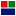Buck model within the project.

5. Ensure that the PWM switching frequency in Device configuration page in project properties, is set to 100 kHz.

6. Ensure that the PWM channel is set to the Si power pole, i.e. Channel is set to 1.

##### Preparing the setup

This setting is exactly same as the one used earlier in Buck converter - Continuous conduction mode (CCM).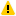Warning

Before proceeding, ensure that the isolated power supply is powered down and that the USB cable is disconnected.

1. Magnetics card connection: Unscrew any existing magnetics card and replace it with the Buck/Boost/Buck-boost magnetics card. Prior to connecting the card, ensure that the jumper on the bottom of the magnetics card is removed. Make sure that all the 6-pins of the magnetics card are making solid contact with the power-pole board.Warning

Never leave the magnetics card unscrewed. If contact is lost while the converter is running, it has the potential to cause very high voltage due to interruption of inductor current, potentially damaging the converter, or worse, could lead to a safety hazard.

2. Rheostat setting: Set the slider such that the resistance across the two closest rheostat terminals is 10 Ω.

3. Power connections:

1. Connect the Vin terminals to the isolated power supply:

1. DC +ve: Vin+ (Red)

2. Ground: Earth (Green)

3. `DC −ve: ● Vin− (Black)`

2. Connect the Vo terminals to the rheostat load:

1. Rheostat rail: Vo+ (Red)

2. `Rheostat's other terminal that is closest to the rail: ● Vo− (Black)`

4. DSO connections:

1. Connect DSO channel 1 probe to Io. Set the following options, if they are supported by the DSO:

1. Set the probe to 1x.

2. Set the measurement type as Current.

3. Set the scaling factor to 5x.

4. Set the offset at 1.5 V or 7.5 A.

5. Set termination to 1 MHz.

6. Set the channel as inverted.

2. Connect DSO channel 2 probe to SWA. Set the following options, if they are supported by the DSO:

1. Set the probe to 1x.

2. Set the measurement type as Voltage.

3. Set the scaling factor to 1x.

4. Set the offset at 0 V.

5. Set termination to 1 MHz.

6. Set the channel as default/non-inverted.

3. Connect DSO channel 3 probe to Iin. Use the same setting as that of Channel 1.

4. Connect DSO channel 4 probe to Vout. Use the same setting as that of Channel 2.

5. Jumper settings:

1. Insert the Vdc jumper.

2. Insert the GaN/Si jumper to the Si side.

3. Insert the GaN switch's external diode jumper, labelled "Diode".

4. The jumper on the bottom of the magnetics card was removed in Step 1, while inserting the card.

6. Connect the USB cable to the power-pole board and the computer.

7. DC power supply settings:

1. Make sure that the DC power supply is fully turned down to 0 V prior to turning on the supply.

2. Turn on the power supply and gradually ramp up the voltage from 0 V to 15 V.

3. If the option is available, set the power supply current limit at 4.5 A.

The final wiring should look similar to this: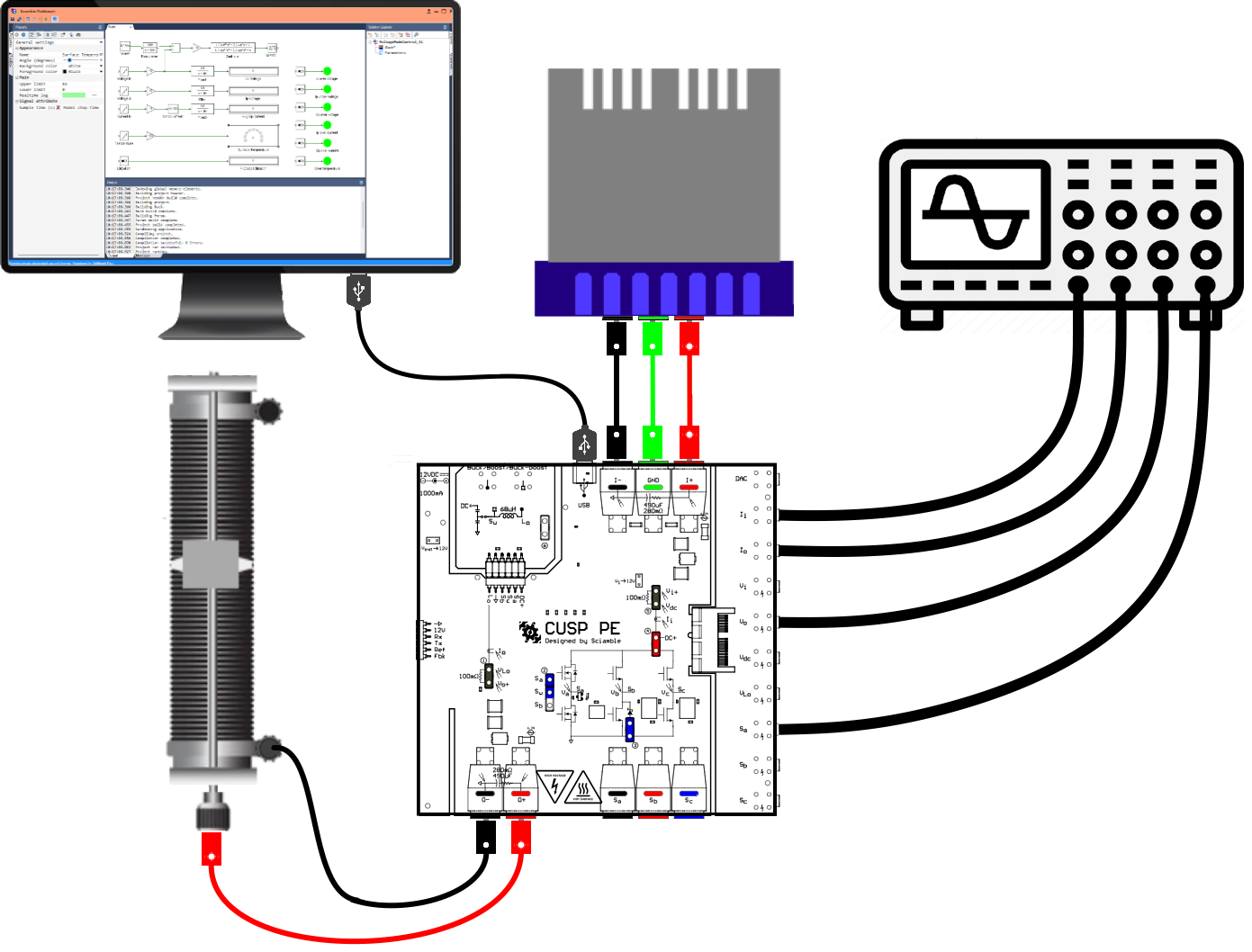##### Real-time open loop control of buck converter - varying load resistance
Running the setup:
1. Click on the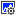icon in the top dock of Workbench to transition from the simulation mode to the real-time mode.

2. Click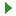to run the control algorithm in real-time.

3. Make note of the current sensor offset.

4. Click on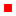to stop the model.

5. Update the values in the Current A and Current B measurement offset.

6. Clickto rerun model.

7. Once programmed, ensure that the Avg Ip Current and the Avg Op Current both initally display a value of 0 for up to 2 decimal places. If not, repeat the previous 3 steps.

8. Gradually increment the duty cycle from 0 to 0.5.

9. Gradually increase the load resistance to the maximum possible value, with at least 9 increments inbetween to make measurements - 4 in CCM, 1 at the border of CCM and DCM, and 4 in DCM.

At the border of CCM and DCM, the inductor current (DSO channel 1), goes to zero just for an instant.

10. Make the following measurements:

1. Make a note of the voltage and current values displayed on Workbench, for each incremental change in the load resistance.

2. Observe the DSO waveforms and make a copy of the voltage across the diode (channel 2), the output voltage (channel 4), the inductor current (channel 1) and the input current (channel 3) for each incremental change in the load resistance. Adjust the time base to show anywhere between 4-10 switching cycles.

3. Under DCM mode, make a note of the frequency of the ringing component in the measured voltage across the diode (channel 2).

11. Click onto stop the model.

12. Repeat this section for switching frequency of 40 kHz.

If required, repeat the same experiment using GaN power-pole instead of Si power-pole as demostrated in Switching characteristic of Si MOSFET/GaN FET and diode.

##### Lab report and reading assignment
1. Attach the DSO waveforms showing the voltage across the diode, the output voltage, the inductor current, and the input current for switching frequency of 100 kHz for varying load resistance.

2. Attach the DSO waveforms showing the voltage across the diode, the output voltage, the inductor current, and the input current for switching frequency of 40 kHz for varying load resistance.

3. For switching frequency of 100 kHz and duty cycle of 0.5, enter the measured values from the Workbench screen capture and calculate the following values:

Vin (V) Vo (V) Io (A) R (Ω) dcalc dact
`        `
`        `
`        `
`        `
`        `
`        `

where, dcalc is 0.5 if in CCM and is given by Eqn. 10 if in DCM. dact = VoVin under both CCM and DCM. Load resistance R = VoIo.

Plot dcalc and dact as a function of the output current Io.

4. For switching frequency of 100 kHz and duty cycle of 0.5, compute the Rcrit using Eqn. 4. Use the inductance value estimated in Buck converter - Continuous conduction mode (CCM) experiment. Compare this result with that of measured value from the above table.

Repeat the same for switching frequency of 40 kHz and duty cycle of 0.5.

5. Using Eqn. 11 and the measured ringing frequency under DCM, estimate the net parasitic capacitance, CD + CP.

##### Reference
1. "Power Electronics, A First Course," Ned Mohan and Siddharth Raju, Wiley Publication.